How Cheenta works to ensure student success?
Explore the Back-Story

# INMO 2013 Question No. 3 Solution3     Letsuch that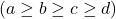. Show that the equation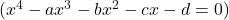has no integer solution.

Sketch of the Solution:

Claim 1: There cannot be a negative integer solution. Suppose other wise. If possible x= -k (k positive) be a solution.

Then we have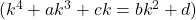. Clearly this is impossible as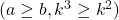and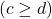.

Claim 2: 0 is not a solution (why?)

Claim 3: There cannot be a positive integer solution. Suppose other wise. If possible x=k (k positive) be a solution.

Then we have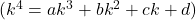This implies that the right hand side is divisible by k which again implies that d is divisible by k (why?).
Let d=d'k
Now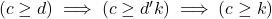.
Thus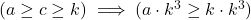.
Hence the equalityis impossible.

3     Letsuch that. Show that the equationhas no integer solution.

Sketch of the Solution:

Claim 1: There cannot be a negative integer solution. Suppose other wise. If possible x= -k (k positive) be a solution.

Then we have. Clearly this is impossible asand.

Claim 2: 0 is not a solution (why?)

Claim 3: There cannot be a positive integer solution. Suppose other wise. If possible x=k (k positive) be a solution.

Then we haveThis implies that the right hand side is divisible by k which again implies that d is divisible by k (why?).
Let d=d'k
Now.
Thus.
Hence the equalityis impossible.

This site uses Akismet to reduce spam. Learn how your comment data is processed.

### Knowledge Partner